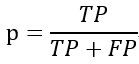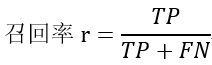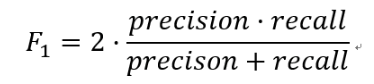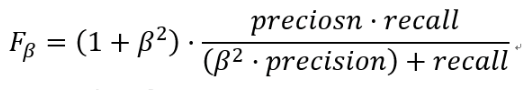8 篇文章 0 订阅

# F1-Score相关概念

• F1分数（F1 Score），是统计学中用来衡量二分类（或多任务二分类）模型精确度的一种指标。它同时兼顾了分类模型的准确率和召回率。F1分数可以看作是模型准确率和召回率的一种加权平均，它的最大值是1，最小值是0，值越大意味着模型越好。假如有100个样本，其中1个正样本，99个负样本，如果模型的预测只输出0，那么正确率是99%，这时候用正确率来衡量模型的好坏显然是不对的。

• 查准率（precision），指的是预测值为1真实值也为1的样本在预测值为1的所有样本中所占的比例。以西瓜问题为例，算法挑出来的西瓜中有多少比例是好西瓜。• 召回率（recall），也叫查全率，指的是预测值为1真实值也为1的样本在真实值为1的所有样本中所占的比例。所有的好西瓜中有多少比例被算法挑了出来。• F1分数（F1-Score），又称为平衡F分数（BalancedScore），它被定义为精确率和召回率的调和平均数。更一般的，我们定义Fβ分数为：除了F1分数之外，F0.5分数和F2分数，在统计学中也得到了大量应用，其中，F2分数中，召回率的权重高于精确率，而F0.5分数中，精确率的权重高于召回率。

# Macro-F1和Micro-F1

• Macro-F1和Micro-F1是相对于多标签分类而言的。
• Micro-F1，计算出所有类别总的Precision和Recall，然后计算F1。
• Macro-F1，计算出每一个类的Precison和Recall后计算F1，最后将F1平均。

# tensorflow实现Macro-F1

import tensorflow as tf
def f1(y_hat, y_true, model='multi'):
'''
输入张量y_hat是输出层经过sigmoid激活的张量
y_true是label{0,1}的集和
model指的是如果是多任务分类，single会返回每个分类的f1分数，multi会返回所有类的平均f1分数（Marco-F1）
如果只是单个二分类任务，则可以忽略model
'''
epsilon = 1e-7
y_hat = tf.round(y_hat)#将经过sigmoid激活的张量四舍五入变为0，1输出

tp = tf.reduce_sum(tf.cast(y_hat*y_true, 'float'), axis=0)
#tn = tf.sum(tf.cast((1-y_hat)*(1-y_true), 'float'), axis=0)
fp = tf.reduce_sum(tf.cast(y_hat*(1-y_true), 'float'), axis=0)
fn = tf.reduce_sum(tf.cast((1-y_hat)*y_true, 'float'), axis=0)

p = tp/(tp+fp+epsilon)#epsilon的意义在于防止分母为0，否则当分母为0时python会报错
r = tp/(tp+fn+epsilon)

f1 = 2*p*r/(p+r+epsilon)
f1 = tf.where(tf.is_nan(f1), tf.zeros_like(f1), f1)
if model == 'single':
return f1
if model == 'multi':
return tf.reduce_mean(f1)


# 测试

• 测试变量是多任务分类的输出
import tensorflow as tf

y_true = tf.constant([[1,1,0,0,1], [1,0,1,1,0], [0,1,1,0,0]])
y_hat = tf.constant([[0,1,1,1,1], [1,0,0,1,1], [1,0,1,0,0]])

with tf.Session() as sess:
f1 = f1(y_hat, y_true)
print('F1 score:', sess.run(f1))

F1 score: 0.5999999


# numpy实现Macro-F1

（2019.1.12更新）

import numpy as np
def f1(y_hat, y_true, THRESHOLD=0.5):
'''
y_hat是未经过sigmoid函数激活的
输出的f1为Marco-F1
'''
epsilon = 1e-7
y_hat = y_hat>THRESHOLD
y_hat = np.int8(y_hat)
tp = np.sum(y_hat*y_true, axis=0)
fp = np.sum(y_hat*(1-y_true), axis=0)
fn = np.sum((1-y_hat)*y_true, axis=0)

p = tp/(tp+fp+epsilon)#epsilon的意义在于防止分母为0，否则当分母为0时python会报错
r = tp/(tp+fn+epsilon)

f1 = 2*p*r/(p+r+epsilon)
f1 = np.where(np.isnan(f1), np.zeros_like(f1), f1)

return np.mean(f1)


# 参考资料

 https://www.kaggle.com/guglielmocamporese/macro-f1-score-keras
 分类问题的几个评价指标（Precision、Recall、F1-Score、Micro-F1、Macro-F1）

08-01535208-2672
09-163411
12-30595
12-19609
05-104354
11-05690
01-02317
08-29342
07-223061
05-1010万+
08-212353
12-059084
09-053822
12-28774
08-28474
07-041万+
08-225152点击重新获取扫码支付余额充值